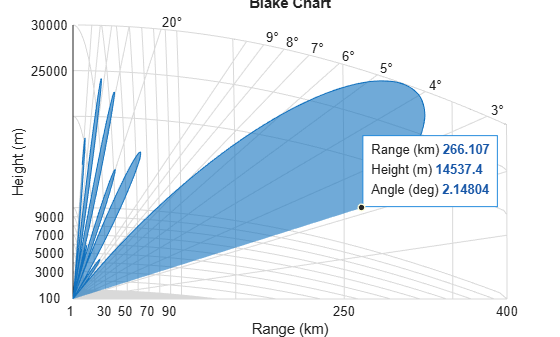The point target radar range equation estimates the power at the input to the receiver for a target of a given radar cross section at a specified range. In this equation, the signal model is assumed to be deterministic. The equation for the power at the input to the receiver is

`${P}_{r}=\frac{{P}_{t}{G}_{t}{G}_{r}{\lambda }^{2}\sigma }{{\left(4\pi \right)}^{3}{R}_{t}^{2}{R}_{r}^{2}L},$`

where the terms in the equation are:

• Pr — Received power in watts.

• Pt — Peak transmit power in watts.

• Gt — Transmitter gain.

• λ — Radar operating frequency wavelength in meters.

• σ — Target's nonfluctuating radar cross section in square meters.

• L — General loss factor to account for both system and propagation loss.

• Rt — Range from the transmitter to the target.

• Rr — Range from the receiver to the target. If the radar is monostatic, the transmitter and receiver ranges are identical.

Sometimes the radar equation is written to include a pattern propagation factor F which takes into account the effects of wave propagation in a non-free space environment where constructive or destructive interference can occur. While the radar equation presented here does not include this term, it can be easily included in the general loss factor, L, by replacing L by L/F. Omitting this term is equivalent to setting F = 1.

`${P}_{r}=\frac{{P}_{t}{G}_{t}{G}_{r}{\lambda }^{2}\sigma F}{{\left(4\pi \right)}^{3}{R}_{t}^{2}{R}_{r}^{2}L}.$`

The equation for the power at the input to the receiver represents the signal term in the signal-to-noise ratio (SNR). To model the noise term, assume the thermal noise in the receiver has a white noise power spectral density (PSD) given by

`$P\left(f\right)=kT,$`

where k is the Boltzmann constant and T is the effective noise temperature. The receiver acts as a filter to shape the white noise PSD. Assume that the magnitude squared receiver frequency response approximates a rectangular filter with bandwidth equal to the reciprocal of the pulse duration, 1/τ. The total noise power at the output of the receiver is

`$N=\frac{kT{F}_{n}}{\tau },$`

where Fn is the receiver noise figure.

The product of the effective noise temperature and the receiver noise factor is referred to as the system temperature and is denoted by Ts, so that Ts = TFn .

Using the equation for the received signal power and the output noise power, the receiver output SNR is

`$\frac{{P}_{r}}{N}=\frac{{P}_{t}\tau \text{​}\text{ }{G}_{t}{G}_{r}{\lambda }^{2}\sigma }{{\left(4\pi \right)}^{3}k{T}_{s}{R}_{t}^{2}{R}_{r}^{2}L}.$`

Solving for the required peak transmit power,

`${P}_{t}=\frac{{P}_{r}{\left(4\pi \right)}^{3}k{T}_{s}{R}_{t}^{2}{R}_{r}^{2}L}{N\tau {G}_{t}{G}_{r}{\lambda }^{2}\sigma }.$`

### Plot Vertical Coverage Pattern Using Default Parameters

Set the frequency to 100 MHz, the antenna height to 10 m, and the free-space range to 200 km. The antenna pattern, surface roughness, antenna tilt angle, and field polarization assume their default values as specified in the `AntennaPattern`, `SurfaceRoughness`, `TiltAngle`, and `Polarization` properties.

Obtain an array of vertical coverage pattern values and angles.

```freq = 100e6; ant_height = 10; rng_fs = 200; [vcp,vcpangles] = radarvcd(freq,rng_fs,ant_height);```

To see the vertical coverage pattern, omit the output arguments.

`radarvcd(freq,rng_fs,ant_height);`### Compute Peak Power Using Radar Equation Calculator App

The `radarEquationCalculator` app lets you determine key radar characteristics such as detection range, required peak transmit power, and SNR. The App works for monostatic and bistatic radars.

When you type `radarEquationCalculator` from the command line or select the app from the App Toolstrip, an interactive window opens. The default window shows a calculation of target range from SNR, power, and other parameters. You can then select various options to compute different radar parameters.

`radarEquationCalculator`Compute Required Peak Transmit Power of Monostatic Radar

As an example, use the app to compute the required peak transmit power for a monostatic radar to detect a large target at 100 km. The radar operates at 10 GHz with a 40 dB antenna gain. Set the probability of detection to 0.9 and the probability of false alarm to 0.0001.

1. From the Calculation Type drop-down list, choose `Peak Transmit Power`

2. Set the Wavelength to `3` cm

3. Specify the Pulse Width as `2` microseconds

4. Assume total System Losses of `5` dB

5. Assuming the target is a large airplane, set Target Radar Cross Section value to `100` ${\mathrm{m}}^{2}$

6. Choose Configuration as `Monostatic`

7. Set the Gain to be `40` dB

8. Open the SNR box

9. Specify the Probability of Detections as `0.9`

10. Specify the Probability of False Alarm as `0.0001`

The app shows that the required peak transmit power is 0.2095 W.